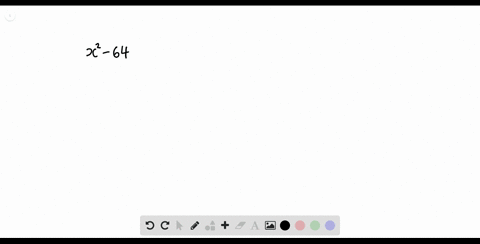Enroll in one of our FREE online STEM summer camps. Space is limited so join now!View Summer Courses### Factor each polynomial. If a polynomial cannot be…

00:35Other Schools

Need more help? Fill out this quick form to get professional live tutoring.

Get live tutoring
Problem 20

Factor each polynomial. If a polynomial cannot be factored, write prime. Factor out the greatest common factor as necessary.
$$24 x^{4}+36 x^{3} y-60 x^{2} y^{2}$$

$24 x^{4}+36 x^{3} y-60 x^{2} y^{2}=12 x^{2}(x-y)(2 x+5 y)$

## Discussion

You must be signed in to discuss.

## Video Transcript

were asked a factor. This train a meal here, Step one. We try to find a common factor between all three terms. Now we can see we can factor out of 12 and an ex great. So once we do that, we're left with two X squared plus three x y minus five y squared. So now we can see that we have a try. No meal here where the leading coefficient is not one. So we need to do isn't going to take the two and the negative five and multiply them together, which we get naked of 10. So we want to find two factors of negative 10 that multiply together to be negative. 10. Add together to be positive three. So that would be positive five times negative too. So once we find that we're going to rewrite our try no meal. But we're going to split up the middle term so we will have call X skirt in front still, and then this turns into two ex curb plus by of X y minus to X Y minus five y skirt. Now, once we have that, we're going to split this up into who turns out of time, and we will try to factor out what we can from each hairs of turns. So from the two extra plus five x y, we can only factor out the next. Now we're left with two X plus five wives. We found these next two terms we can factor out the negative. Why, no more left was two X plus five wine. So now we can see that these two terms both have the two X plus five. So that's a common factor. So that means we can factor that out. We could 12 x squared. You're factor out the two X plus five. By now we're left with X minus y. So do toothy associative property of multiplication. We actually don't need this big bracket here, so we can just get rid of that. So answer is now 12 X squared times two x plus Bye bye times X minus Y